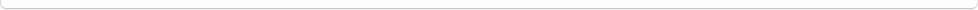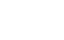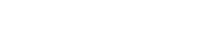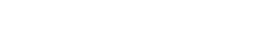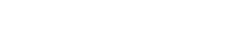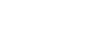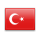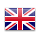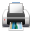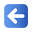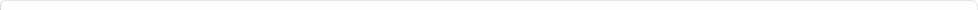Description of Individual Course Units
 Course Unit Code Course Unit Title Type of Course Unit Year of Study Semester Number of ECTS Credits 190400706106 STATISTIC II Elective 3 6 3
Level of Course Unit
First Cycle
Objectives of the Course
Data to make statistical evaluations, review, existence, basic accounts and formulations
Name of Lecturer(s)
Learning Outcomes
 1 To be able to define the properites of estimators and importance in the theory of statistical estimation 2 To be able to define the properites of estimators and importance in the theory of statistical estimation 3 To be able to define the populations according to the representative sample taken 4 To be able to define the populations according to the representative sample taken 5 To be able to establish the relationship between probability distributions and hypothesis testing 6 To be able to establish the relationship between probability distributions and hypothesis testing 7 To be able to propose appropriate regression models the dependent and independent variables 8 To be able to propose appropriate regression models the dependent and independent variables
Mode of Delivery
Daytime Class
Prerequisites and co-requisities
-
Recommended Optional Programme Components
-
Course Contents
Statistical estimation, hypothesis testing, chi-square test, analysis of correlation and regression, analysis of variance and indexes.
Weekly Detailed Course Contents
 Week Theoretical Practice Laboratory 1 Confidence Intervals: For populations means , population is normal :population variance is known, Student t distribution, For populations means , population is normal :population variance is not known, For population proprotions. - - 2 Chi-spuare distribution, Confidence interval for population variance, population are normal, Confidence inreval for the difference of two populations means , populations are normal, Confidence interval for paired samples which are normal, Confidence interval for the difference of two independent sample means - - 3 Confidence interval for the difference of two populations proprotions. (large samples), Sample size for the confidence interval for population means , population is normal, population variance is known, samples size for the confidence interval for the population proprotion. - - 4 Hypothesis Testing: basic consepts, hypothesis testing for normal population mean: population variance is known, hypothesis testing for normal population mean: population variance is not known, ( small and large samples ), hypothesis testing for population proportion( large samples) - - 5 Hypothesis testing for normal population variance, hypothesis testing for two populations means: Paired Saples, Independent Samples, hypothesis testing for two population proportions, F distibution, hypothesis testing of two populations variances, calculation of type II error - - 6 Chi- square goodness of fits test ( uniform, binom, poisson and normal),rxc independent test - - 7 Chi- square goodness of fits test ( uniform, binom, poisson and normal),rxc independent test - - 8 Regression analysis: Simple linear regression, mean square estimation, the assumptions of linear regression - - 9 Analysis of variance for regression model, the estimation of coefficient of deternination and significant test, the estimation of correlaiton coefficient and significant test - - 10 One way and two way anova ( analysis of variance ) - - 11 Index Number : A simple index, Chain Index, Time and Space Indexes, Fixed Based Index, Variable-Based Index, an index to the other transition, main (base) Circuit Identification, indexes Average, Weighted Indexes, Some Important Indexes - - 12 Time Series Analysis and Estimating: A Time Series Components, Moving Averages, Determination of the Effect of Seasonal Using Moving Averages - - 13 Simple random sampling, Stratified sampling, cluster sampling, systematic sampling Introducing the Social Sciences Applications - - 14 Simple random sampling, Stratified sampling, cluster sampling, systematic sampling Introducing the Social Sciences Applications - -
Planned Learning Activities and Teaching Methods
Assessment Methods and Criteria
 Term (or Year) Learning Activities Quantity Weight Midterm Examination 1 100 SUM 100 End Of Term (or Year) Learning Activities Quantity Weight Final Examination 1 100 SUM 100 Term (or Year) Learning Activities 40 End Of Term (or Year) Learning Activities 60 SUM 100
Language of Instruction
Turkish
Work Placement(s)
-